Courses

# Test: Analysis of Steam Cycles - 3

## 30 Questions MCQ Test Topicwise Question Bank for Mechanical Engineering | Test: Analysis of Steam Cycles - 3

Description
This mock test of Test: Analysis of Steam Cycles - 3 for Mechanical Engineering helps you for every Mechanical Engineering entrance exam. This contains 30 Multiple Choice Questions for Mechanical Engineering Test: Analysis of Steam Cycles - 3 (mcq) to study with solutions a complete question bank. The solved questions answers in this Test: Analysis of Steam Cycles - 3 quiz give you a good mix of easy questions and tough questions. Mechanical Engineering students definitely take this Test: Analysis of Steam Cycles - 3 exercise for a better result in the exam. You can find other Test: Analysis of Steam Cycles - 3 extra questions, long questions & short questions for Mechanical Engineering on EduRev as well by searching above.
QUESTION: 1

### For a mercury-steam-sulphur dioxide cycle, the heat rejected in the mercury cycle is given to the steam cycle and the heat rejected in the steam cycle is utilized in the SO2 cycle. If the efficiencies of the mercury, steam and SO2 cycles are 0.52,0.41 and 0.25 respectively, what is the overall efficiency of the composite cycle?

Solution:

η = 1 - (1 - η1) (1 - η2) (1 - η3)
= 1 - (1 - 0.52) (1 - 0.41) (1 - 0 .2 5)
= 1 - 0.48 x 0.59 x 0.75 = 0.7876

QUESTION: 2

### Consider the following advantages of combined gas-steam cycles: 1. High overall plant efficiency 2. Low investment cost 3. Small amount of water required 4. Quick start-up but slow shut-down Q. Which of the above statements are correct?

Solution:

- High overall plant efficiency
- Low investment cost
- Small amount of water required
- Quick shut-down and start-up of the plant
- Phased installation
- Simplicity of operation
- Low environmental impact
- Advantages for cogeneration of heat and electricity.

QUESTION: 3

### Heating of dry steam above saturation temperature is known as

Solution: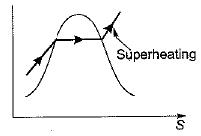QUESTION: 4

At critical point the enthalpy of vaporization is

Solution:

As pressure increases, the enthalpy of vaporization decreases and at critical point saturated water directly converted into saturated steam hence enthalpy of vaporization is zero at critical point.

QUESTION: 5

When saturation pressure of water vapour increases

Solution:

As saturation pressure increases, enthalpy of vaporization decreases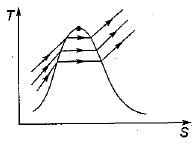QUESTION: 6

The correct representation of a simple Rankine cycle on a T-S diagram is

Solution:

Rankine Cycle: Ranking cycle consists of two isentropic process and two reversible isobaric process.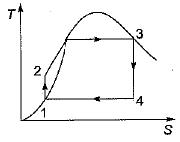QUESTION: 7

In the Rankine cycle, the heat rejection process takes place at constant

Solution:

In Rankine cycle heat reject on takes place at constant pressure.

QUESTION: 8

A superheat Rankine Cycle is shown in the given T - S diagram. Starting from the feed pump, the fluid flow upto the boiler exit is represented by straight-line.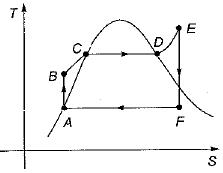Solution: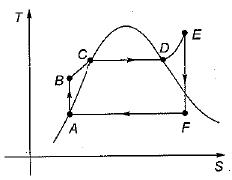In the Rankine cycle
AB ⇒ isentropic compression (Feed pump)
BE ⇒ Constant pressure Heat addition (In Boiler)
EF ⇒ Isentropic expansion (In turbine)
FA ⇒ Constant pressure heat rejection (In condenser)
Hence cycle is A - B - C - D - E is cycle
Starting from feed pump up to boiler exit.

QUESTION: 9

In the Rankine cycle the lower limit on the condenser pressure is due to the

Solution:
QUESTION: 10

Reheating of steam under ideal condition takes place at constant

Solution: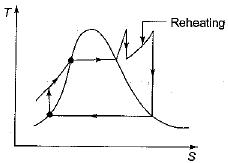Reheating is the constant pressure heat addition process after expanding steam in high pressure turbine.

QUESTION: 11

Employing superheated steam in turbine leads to

Solution:

Superheating of steam leads to decrease in erosion of turbine blades and increase in the fife of turbine blades.

QUESTION: 12

The reheat cycle in steam power plant is mainly adopted to

Solution:

Reheating improves the quality of steam at the exit of turbine.

QUESTION: 13

The curve labelled 1 - 2 in the given figure refers to the expansion process of a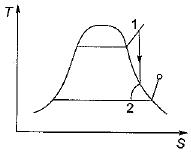Solution:
QUESTION: 14

What is the difference between the temperature of feed water outlet and saturation temperature of steam entering the heater called?

Solution:
QUESTION: 15

Consider an actual regenerative Rankine cycle with one open feed water heater. For each kg steam entering the turbine, if m kg steam with a specific enthalpy of h1 is bleed from the turbine, and the specific enthalpy of liquid water entering the heater is h2, then h3 specific enthalpy of saturated liquid leaving the heater is equal to

Solution:

By analyzing input and output energy on open feed water heater, we get
h3 = mh1 + (1 - m)h2 = mh1 + h2- mh2
h3 = h2 - m (h- h1)

QUESTION: 16

For a given sot of operating pressure limits of a Rankine cycle, the highest efficiency occurs for

Solution:

Efficiency of ideal regenerative cycle is exactly equal to that of the corresponding Carnot cycle. Hence it is maximum.

QUESTION: 17

A steam plant has the boiler efficiency of 92%, turbine efficiency (mechanical) of 94%, generator efficiency of 95% and cycle efficiency of 44%. If 6% of the generated power is used to run the auxiliaries the overall plant efficiency is

Solution: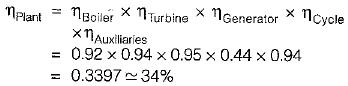QUESTION: 18

Stirling cycle and a Carnot cycle operate between 50° and 350°C. Their efficiencies are ηand ηc respectively. In this case, which of the following statements is true?

Solution:

Efficiency of Stirling cycle is equal to Carnot cycle only when regenerative arrangement is employed.

QUESTION: 19

The efficiency of superheat Rankine cycle is higher than that of simple Rankine cycle because

Solution:

Due to higher mean temperature of heat addition of superheat Rankine cycle its efficiency in higher than simple Rankine cycle.

QUESTION: 20

Which one of the following cycles working within the same temperature limits has the highest work ratio?

Solution:

Work ratio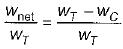Due to use of pump in Rankine cycle, its work ratio is very high as compared to Carnot. Pump work is very small.

QUESTION: 21

When two binary vapour cycles (with topping cycle and bottoming cycle) are coupled in series, what is the overall efficiency?

Solution:

Overall efficiency of binary vapour cycle is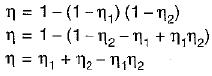QUESTION: 22

For a mercury-steam sulphur-dioxide cycle, the heat rejected in the mercury cycle is given to the steam cycle and the heat rejected in the steam cycle is utilized in SO2 cycle. If the efficiencies of the mercury, steam and SO2 cycle 0.5, 0.4 and 0.25 respectively, then overall efficiency of the composite cycle is

Solution:

For 3 cycles coupled in series the overall efficiency of the combined cycle is given by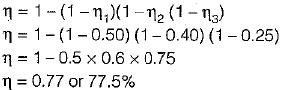QUESTION: 23

The efficiency of Carnot cycle may be equal to any one of the following cycles running between same two temperature limits. Which one is it?

Solution:
QUESTION: 24

In a simple Rankine cycle, steam enters the condenser at saturated vapour and leaves as saturated liquid. The condenser the boiler pressures are maintained at ρ1 and ρ2, respectively. If vf and vg are the specific volumes of saturated liquid and saturated vapour at condenser pressure, then pump work can be approximated as

Solution:
QUESTION: 25

The ideal cycle for a steam power plant is the Rankine cycle instead of the Carnot cycle because

Solution:
QUESTION: 26

The adiabatic enthalpy drop across the prime mover of the Rankine cycle is 840 kJ/kg. The enthalpy of steam supplied is 294 kJ/kg. If the back pressure is 0.1 bar the specific steam consumption is lf hf at 0.1 bar = 191.8 kJ/kg.

Solution:

Useful work done = 840 kJ/Kg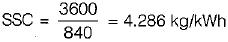QUESTION: 27

Group-I shows different heat addition processes in power cycle. Like wise Group-II shows different heat removal processes. Group-Ill lists Power cycles.
Match item from Group I, II and III
Group-I
P-Pressure const.
Q-Volume const.
R-Temp Const.
Group-II
S-Pressure Const.
T-Volume const.
U-Temp const.
Group-Ill
1. Rankine cycle
2. Otto cycle
3. Carnot cycle
4. Diesel cycle
5. Brayton cycle

Solution:
QUESTION: 28

Consider the following statements:
The efficiency of the vapour power Rankine cycle can increased by
1. Increasing the temperature of the working fluid at which heat is added
2. Increasing the pressure of the working fluid at which heat is added
3. Decreasing the temperature of the working fluid at which heat is reject
Q. Which of these statements is/are correct?

Solution: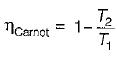Where T2 is the temperature of the rejection and T1, is the temperature of heat added. Efficiency increases when T2 decreases and T1, increases. An increases in the pressure of working fluid increases the mean temperature of heat addition and hence increases the cycle efficiency.

QUESTION: 29

The enthalpy of steam entering a turbine in a Rankine cycle is 200 kJ/kg. The enthalpy after isentropic expansion is 2400 kJ/kg and the enthalpy at the end of actual expansion is 2560 kJ/kg. What is the turbine efficiency?

Solution:

Turbine efficiency (η)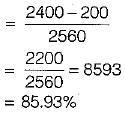QUESTION: 30

Consider the following statements regarding superheating in Rankine Cycles:
1. it reduces the specific steam consumption.
2. it increase the dryness fraction of steam at the exhaust for the same value of condenser pressure.
3. It reduces the cycle efficiency
Of these statements:

Solution:

Due to increase in net workout put specific steam consumption decreases and due to reheatin dryness fraction at the exit of turbine improves.